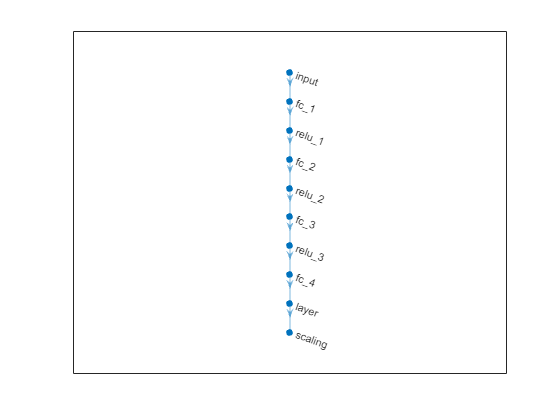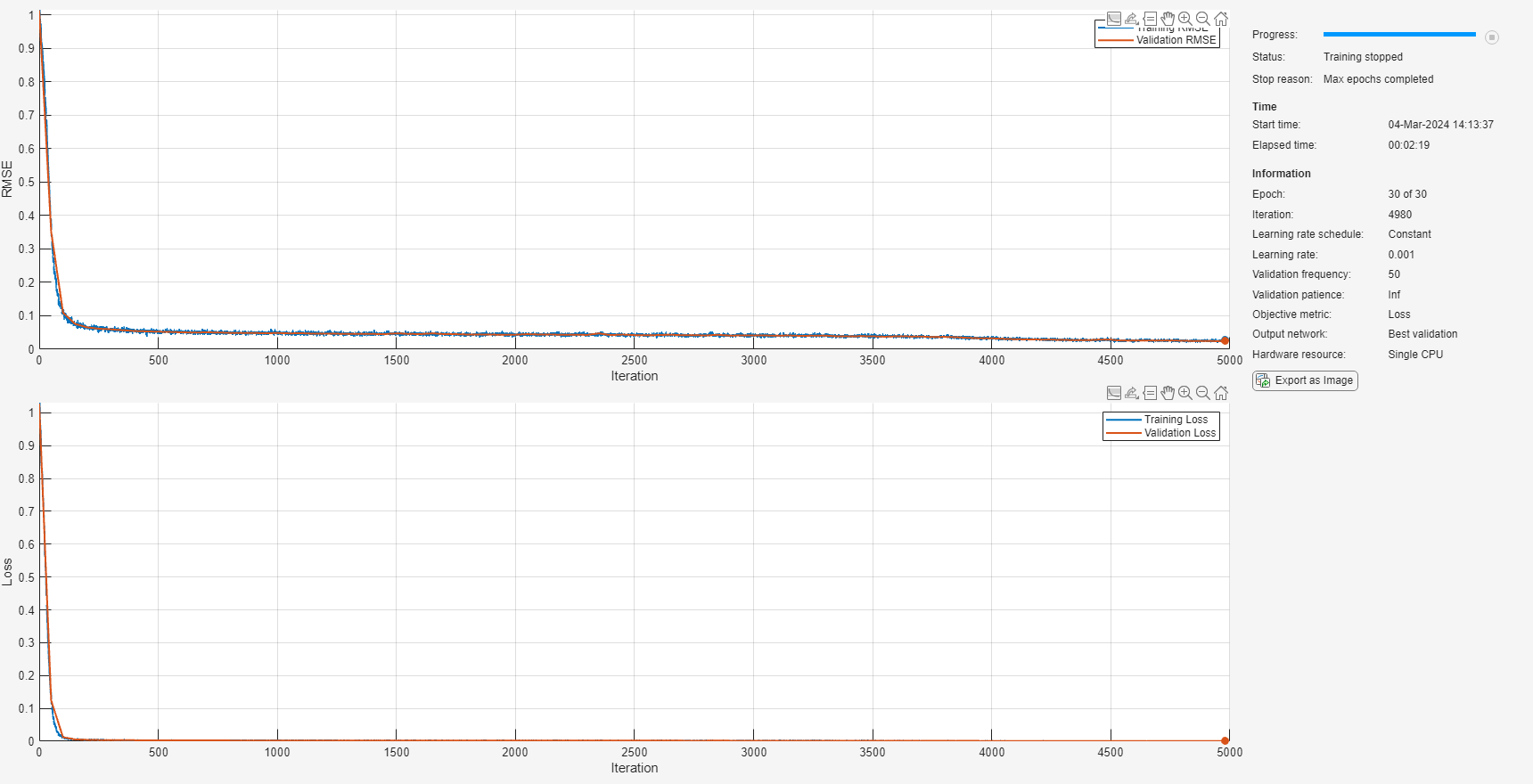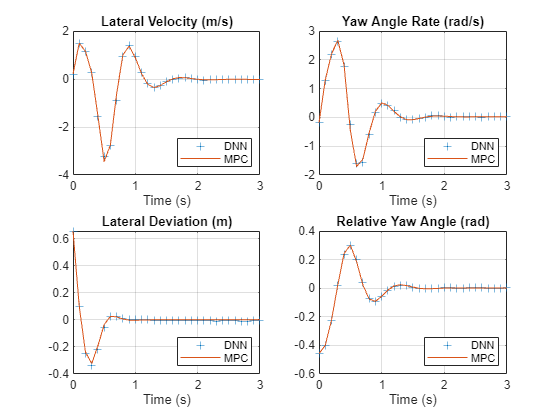# Imitate MPC Controller for Lane Keeping Assist

This example shows how to train, validate, and test a deep neural network that imitates the behavior of a model predictive controller for an automotive lane keeping assist system. In the example, you also compare the behavior of the deep neural network with that of the original controller.

Model predictive control (MPC) solves a constrained quadratic-programming (QP) optimization problem in real time based on the current state of the plant. Because MPC solves its optimization problem in an open-loop fashion, you can potentially replace the controller with a deep neural network. Evaluating a deep neural network is more computationally efficient than solving a QP problem in real time.

If the training of the network sufficiently traverses the state-space for the application, you can create a reasonable approximation of the controller behavior. You can then deploy the network for your control application. You can also use the network as a warm starting point for training the actor network of a reinforcement learning agent. For an example, see Train DDPG Agent with Pretrained Actor Network.

### Design MPC Controller

Design an MPC controller for lane keeping assist. To do so, first create a dynamic model for the vehicle.

`[sys,Vx] = createModelForMPCImLKA;`

Create and design the MPC controller object `mpcobj`. Also, create an `mpcstate` object for setting the initial controller state. For details on the controller design, type `edit createMPCobjImLKA`.

`[mpcobj,initialState] = createMPCobjImLKA(sys);`

For more information on designing model predictive controllers for lane keeping assist applications, see Lane Keeping Assist System Using Model Predictive Control (Model Predictive Control Toolbox) and Lane Keeping Assist with Lane Detection (Model Predictive Control Toolbox).

### Prepare Input Data

Load the input data from `InputDataFileImLKA.mat`. The columns of the data set follow:

1. Lateral velocity ${\mathit{V}}_{\mathit{y}}$

2. Yaw angle rate $\mathit{r}$

3. Lateral deviation ${\mathit{e}}_{1}$

4. Relative yaw angle ${\mathit{e}}_{2}$

5. Previous steering angle (control variable) $\mathit{u}$

6. Measured disturbance (road yaw rate: longitudinal velocity * curvature ($\rho$))

7. Cost function value

8. MPC iterations

9. Steering angle computed by MPC controller: ${\mathit{u}}^{*}$

The data in `InputDataFileImLKA.mat` was created by computing the MPC control action for randomly generated states, previous control actions, and measured disturbances. To generate your own training data, use the `collectDataImLKA` function.

```dataStruct = load('InputDataFileImLKA.mat'); data = dataStruct.Data;```

Divide the input data into training, validation, and testing data. First, determine the number of validation data rows based on a given percentage.

```totalRows = size(data,1); validationSplitPercent = 0.1; numValidationDataRows = floor(validationSplitPercent*totalRows);```

Determine the number of test data rows based on a given percentage.

```testSplitPercent = 0.05; numTestDataRows = floor(testSplitPercent*totalRows);```

Randomly extract validation and testing data from the input data set. To do so, first randomly extract enough rows for both data sets.

```randomIdx = randperm(totalRows,numValidationDataRows + numTestDataRows); randomData = data(randomIdx,:);```

Divide the random data into validation and testing data.

```validationData = randomData(1:numValidationDataRows,:); testData = randomData(numValidationDataRows + 1:end,:);```

Extract the remaining rows as training data.

```trainDataIdx = setdiff(1:totalRows,randomIdx); trainData = data(trainDataIdx,:);```

Randomize the training data.

```numTrainDataRows = size(trainData,1); shuffleIdx = randperm(numTrainDataRows); shuffledTrainData = trainData(shuffleIdx,:);```

Reshape the training and validation data into 4-D matrices for use with `trainNetwork`.

```numObservations = 6; numActions = 1; trainInput = shuffledTrainData(:,1:6); trainOutput = shuffledTrainData(:,9); validationInput = validationData(:,1:6); validationOutput = validationData(:,9); validationCellArray = {validationInput,validationOutput};```

Reshape the testing data for use with `predict`.

```testDataInput = testData(:,1:6); testDataOutput = testData(:,9);```

### Create Deep Neural Network

The deep neural network architecture uses the following layers.

• `imageInputLayer` is the input layer of the neural network.

• `fullyConnectedLayer` multiplies the input by a weight matrix and then adds a bias vector.

• `reluLayer` is the activation function of the neural network.

• `tanhLayer` constrains the value to the range to [-1,1].

• `scalingLayer` scales the value to the range to [-1.04,1.04], implies that the steering angle is constrained to be [-60,60] degrees.

• `regressionLayer` defines the loss function of the neural network.

Create the deep neural network that will imitate the MPC controller after training.

```imitateMPCNetwork = [ featureInputLayer(numObservations,'Normalization','none','Name','InputLayer') fullyConnectedLayer(45,'Name','Fc1') reluLayer('Name','Relu1') fullyConnectedLayer(45,'Name','Fc2') reluLayer('Name','Relu2') fullyConnectedLayer(45,'Name','Fc3') reluLayer('Name','Relu3') fullyConnectedLayer(numActions,'Name','OutputLayer') tanhLayer('Name','Tanh1') scalingLayer('Name','Scale1','Scale',1.04) regressionLayer('Name','RegressionOutput') ];```

Plot the network.

`plot(layerGraph(imitateMPCNetwork))`### Train Deep Neural Network

Specify training options.

```options = trainingOptions('adam', ... 'Verbose',false, ... 'Plots','training-progress', ... 'Shuffle','every-epoch', ... 'MaxEpochs', 30, ... 'MiniBatchSize',512, ... 'ValidationData',validationCellArray, ... 'InitialLearnRate',1e-3, ... 'GradientThresholdMethod','absolute-value', ... 'ExecutionEnvironment','cpu', ... 'GradientThreshold',10, ... 'Epsilon',1e-8);```

Train the deep neural network. To view detailed training information in the Command Window, set the `'Verbose'` training option to `true`.

`imitateMPCNetObj = trainNetwork(trainInput,trainOutput,imitateMPCNetwork,options);`

Training of the deep neural network stops after the final iteration.

The training and validation loss are nearly the same for each mini-batch, which indicates that the trained network does not overfit.

### Test Trained Network

Check that the trained deep neural network returns steering angles similar to the MPC controller control actions given the test input data. Compute the network output using the `predict` function.

`predictedTestDataOutput = predict(imitateMPCNetObj,testDataInput);`

Calculate the root mean squared error (RMSE) between the network output and the testing data.

```testRMSE = sqrt(mean((testDataOutput - predictedTestDataOutput).^2)); fprintf('Test Data RMSE = %d\n', testRMSE);```
```Test Data RMSE = 3.200035e-02 ```

The small RMSE value indicates that the network outputs closely reproduce the MPC controller outputs.

### Compare Trained Network with MPC Controller

To compare the performance of the MPC controller and the trained deep neural network, run closed-loop simulations using the vehicle plant model.

Generate random initial conditions for the vehicle that are not part of the original input data set, with values selected from the following ranges:

1. Lateral velocity ${\mathit{V}}_{\mathit{y}}$ — Range (-2,2) m/s

2. Yaw angle rate $\mathit{r}$ — Range (-60,60) deg/s

3. Lateral deviation ${\mathit{e}}_{1}$ — Range (-1,1) m

4. Relative yaw angle ${\mathit{e}}_{2}$ — Range (-45,45) deg

5. Last steering angle (control variable) $\mathit{u}$ — Range (-60,60) deg

6. Measured disturbance (road yaw rate, defined as longitudinal velocity * curvature ($\rho$)) — Range (-0.01,0.01) with a minimum road radius of 100 m

```rng(5e7) [x0,u0,rho] = generateRandomDataImLKA(data);```

Set the initial plant state and control action in the `mpcstate` object.

```initialState.Plant = x0; initialState.LastMove = u0;```

Extract the sample time from the MPC controller. Also, set the number of simulation steps.

```Ts = mpcobj.Ts; Tsteps = 30; ```

Obtain the `A` and `B` state-space matrices for the vehicle model.

```A = sys.A; B = sys.B;```

Initialize the state and input trajectories for the MPC controller simulation.

```xHistoryMPC = repmat(x0',Tsteps+1,1); uHistoryMPC = repmat(u0',Tsteps,1);```

Run a closed-loop simulation of the MPC controller and the plant using the `mpcmove` function.

```for k = 1:Tsteps % Obtain plant output measurements, which correspond to the plant outputs. xk = xHistoryMPC(k,:)'; % Compute the next cotnrol action using the MPC controller. uk = mpcmove(mpcobj,initialState,xk,zeros(1,4),Vx*rho); % Store the control action. uHistoryMPC(k,:) = uk; % Update the state using the control action. xHistoryMPC(k+1,:) = (A*xk + B*[uk;Vx*rho])'; end```

Initialize the state and input trajectories for the deep neural network simulation.

```xHistoryDNN = repmat(x0',Tsteps+1,1); uHistoryDNN = repmat(u0',Tsteps,1); lastMV = u0;```

Run a closed-loop simulation of the trained network and the plant. The `neuralnetLKAmove` function computes the deep neural network output using the `predict` function.

```for k = 1:Tsteps % Obtain plant output measurements, which correspond to the plant outputs. xk = xHistoryDNN(k,:)'; % Predict the next move using the trained deep neural network. uk = neuralnetLKAmove(imitateMPCNetObj,xk,lastMV,rho); % Store the control action and update the last MV for the next step. uHistoryDNN(k,:) = uk; lastMV = uk; % Update the state using the control action. xHistoryDNN(k+1,:) = (A*xk + B*[uk;Vx*rho])'; end```

Plot the results, and compare the MPC controller and trained deep neural network (DNN) trajectories.

`plotValidationResultsImLKA(Ts,xHistoryDNN,uHistoryDNN,xHistoryMPC,uHistoryMPC);`The deep neural network successfully imitates the behavior of the MPC controller. The vehicle state and control action trajectories for the controller and the deep neural network closely align.